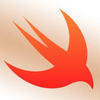## Welcome to the Treehouse Community

Want to collaborate on code errors? Have bugs you need feedback on? Looking for an extra set of eyes on your latest project? Get support with fellow developers, designers, and programmers of all backgrounds and skill levels here with the Treehouse Community!

### Looking to learn something new?

Treehouse offers a seven day free trial for new students. Get access to thousands of hours of content and join thousands of Treehouse students and alumni in the community today.# Stuck

Code Challenge two for Enumeration and Optionals

test.swift
```class Point {
var x: Int
var y: Int

init(x: Int, y: Int) {
self.x = x
self.y = y
}
}

enum Direction {
case left
case right
case up
case down
}

class Robot {
var location: Point

init() {
self.location = Point(x: 0, y: 0)
}

func move(_ direction: Direction) -> Point {
switch direction {
case .up: return Point(x: 0, y: 1)
case .down: return Point(x: 0, y: -1)
case .right: return Point(x: 1, y: 0)
case .left: return Point(x: -1, y: 0)
default: return Point(x: 0, y: 0)
}
}
```Here is how you would complete this challenge; let me know if you need further explanation

```class Point {
var x: Int
var y: Int

init(x: Int, y: Int) {
self.x = x
self.y = y
}
}

enum Direction {
case left
case right
case up
case down
}

class Robot {
var location: Point

init() {
self.location = Point(x: 0, y: 0)
}

func move(_ direction: Direction) {

switch direction {
case .up:
location.y += 1
case .down:
location.y -= 1
case .right:
location.x += 1
case .left:
location.x -= 1

}
}
}
```